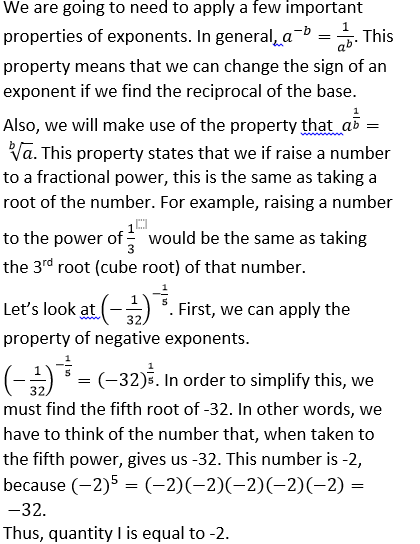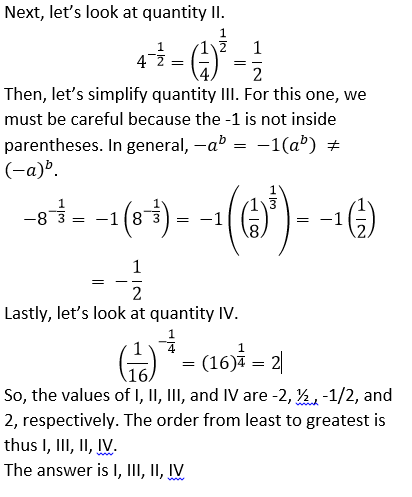## Example Questions

← Previous 1

### Example Question #1 : Exponents And Rational Numbers

Solve for.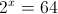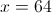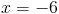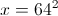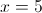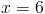Explanation:

Since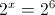Hence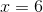### Example Question #2 : Exponents And Rational Numbers

Simplify: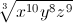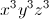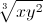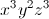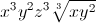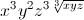Explanation: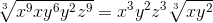### Example Question #3 : Exponents And Rational Numbers

Solve for: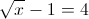Explanation:

From the equation one can see that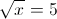Hencemust be equal to 25.

### Example Question #4 : Exponents And Rational Numbers

Evaluate: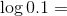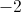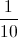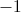Explanation: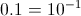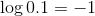### Example Question #5 : Exponents And Rational Numbers

Solve for.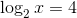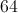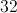Explanation: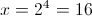### Example Question #1 : Exponents And Rational Numbers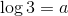and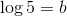Find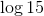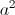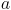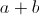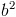Explanation: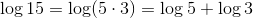Hence the correct answer is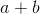.

### Example Question #1 : How To Find An Exponent From A Rational Number

Solve for.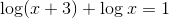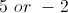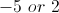Explanation:

Use the rules of logarithms to combine terms.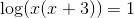Hence,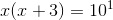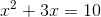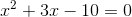By fatoring we get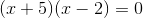Hence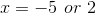.

However, you cannot take the logarithm of a negative number. Thus, the only value foris.

### Example Question #8 : Exponents And Rational Numbers

If a piece of pie is cut into 3 sections, and each of those pieces is further cut into three sections, then those pieces are cut into three sections, how many (tiny) pieces of pie are there?

40

36

125

27

12

27

Explanation:

The answer is 33 = 27

### Example Question #9 : Exponents And Rational Numbers

Solve for.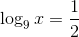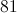Explanation: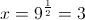### Example Question #231 : Exponents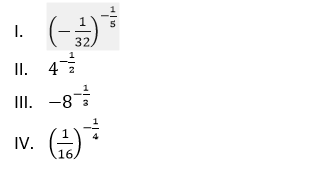Which of the following lists the above quantities from least to greatest?

II, III, I, IV

I, III, II, IV

IV, III, II, I

I, IV, II, III

I, IV, III, II